# High School Math : Understanding Transformation

## Example Questions

### Example Question #2 : Transformations Of Parabolic Functions

If the functionis depicted here, which answer choice graphs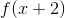?

The function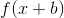shifts a function f(x)units to the left. Conversely,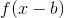shifts a function f(x)units to the right. In this question, we are translating the graph two units to the left.
To translate along the y-axis, we use the function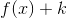or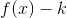.